Courses

# Force between Two Parallel Currents, the Ampere Class 12 Notes | EduRev

## JEE: Force between Two Parallel Currents, the Ampere Class 12 Notes | EduRev

The document Force between Two Parallel Currents, the Ampere Class 12 Notes | EduRev is a part of the JEE Course Physics Class 12.
All you need of JEE at this link: JEE

Consider the system shown in the figure. Here, we have two parallel current carrying conductor, separated by a distance ‘d’, such that one of the conductors is carrying a current I1 and the other is carrying I2, as shown in the figure. From the knowledge gained before, we can say that the conductor 2 experiences the same magnetic field at every point along its length due to the conductor 1. The direction of magnetic force is indicated in the figure and is found using the right-hand thumb rule. The direction of the magnetic field, as we can see, is downwards due to the first conductor.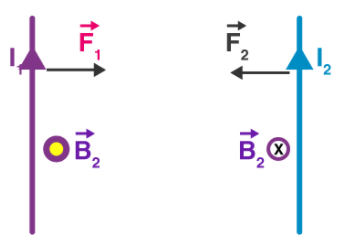Current Carrying conductors

From the Ampere’s circuital law, the magnitude of  the field due to the first conductor can be given by,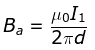The force on a segment of length L of the conductor 2 due to the conductor 1 can be given as,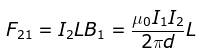Similarly, we can calculate the force exerted by the conductor 2 on the conductor 1. We see that, the conductor 1 experiences the same force due to the conductor 2 but the direction is opposite. Thus,

F12 = F21

We also observe that, the currents flowing in the same direction make the conductors attract each other and that showing in the opposite direction makes the conductors repel each other. The magnitude of force acting per unit length can be given as,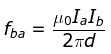The document Force between Two Parallel Currents, the Ampere Class 12 Notes | EduRev is a part of the JEE Course Physics Class 12.
All you need of JEE at this link: JEEUse Code STAYHOME200 and get INR 200 additional OFF Use Coupon Code
All Tests, Videos & Notes of JEE: JEE

### Top Courses for JEE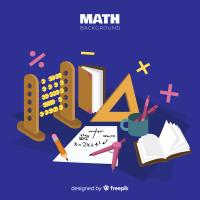## Physics Class 12

132 videos|322 docs|166 tests

### Top Courses for JEETrack your progress, build streaks, highlight & save important lessons and more!

,

,

,

,

,

,

,

,

,

,

,

,

,

,

,

,

,

,

,

,

,

,

,

,

;